https://doi.org/10.5194/acp-20-4367-2020
https://doi.org/10.5194/acp-20-4367-2020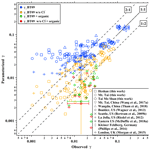# Heterogeneous N2O5 reactions on atmospheric aerosols at four Chinese sites: improving model representation of uptake parameters

Chuan Yu, Zhe Wang, Men Xia, Xiao Fu, Weihao Wang, Yee Jun Tham, Tianshu Chen, Penggang Zheng, Hongyong Li, Ye Shan, Xinfeng Wang, Likun Xue, Yan Zhou, Dingli Yue, Yubo Ou, Jian Gao, Keding Lu, Steven S. Brown, Yuanhang Zhang, and Tao Wang
Abstract

Heterogeneous reactivity of N2O5 on aerosols is a critical parameter in assessing NOx fate, nitrate production, and particulate chloride activation. Accurate measurement of its uptake coefficient (${\mathit{\gamma }}_{{\mathrm{N}}_{\mathrm{2}}{\mathrm{O}}_{\mathrm{5}}}$) and representation in air quality models are challenging, especially in the polluted environment. With an in situ aerosol flow-tube system, the ${\mathit{\gamma }}_{{\mathrm{N}}_{\mathrm{2}}{\mathrm{O}}_{\mathrm{5}}}$ was directly measured on ambient aerosols at two rural sites in northern and southern China. The results were analyzed together with the ${\mathit{\gamma }}_{{\mathrm{N}}_{\mathrm{2}}{\mathrm{O}}_{\mathrm{5}}}$ derived from previous field studies in China to obtain a holistic picture of N2O5 uptake and the influencing factors under various climatic and chemical conditions. The field-derived or measured ${\mathit{\gamma }}_{{\mathrm{N}}_{\mathrm{2}}{\mathrm{O}}_{\mathrm{5}}}$ was generally promoted by the aerosol water content and suppressed by particle nitrate. Significant discrepancies were found between the measured ${\mathit{\gamma }}_{{\mathrm{N}}_{\mathrm{2}}{\mathrm{O}}_{\mathrm{5}}}$ and that estimated from laboratory-determined parameterizations. An observation-based empirical parameterization was derived in the present work, which better reproduced the mean value and variability of the observed ${\mathit{\gamma }}_{{\mathrm{N}}_{\mathrm{2}}{\mathrm{O}}_{\mathrm{5}}}$. Incorporating this new parameterization into a regional air quality model (WRF-CMAQ) has improved the simulation of N2O5, nitrogen oxides, and secondary nitrate in the polluted regions of China.

Share
Dates
1 Introduction

Heterogeneous reaction of dinitrogen pentoxide (N2O5) on aerosol surfaces plays an important role in the nocturnal removal of nitrogen oxides (NOx), secondary nitrate formation, and chlorine activation through nitryl chloride (ClNO2) production on chloride-containing aerosols (Brown et al., 2006; Osthoff et al., 2008; Thornton et al., 2010; Wang et al., 2016). Realistically representing this process in air quality models is therefore necessary for the prediction and mitigation of ground-level ozone and particulate pollution. The currently accepted mechanism of the heterogeneous reaction of N2O5 on aqueous aerosols starts with the mass accommodation of N2O5 on aerosol surface (Reaction R1), followed by reversible N2O5 hydrolysis to form nitrate and intermediate ${\mathrm{H}}_{\mathrm{2}}{\mathrm{ONO}}_{\mathrm{2}}^{+}$ in the aqueous phase (Reaction R2). The intermediate ${\mathrm{H}}_{\mathrm{2}}{\mathrm{ONO}}_{\mathrm{2}}^{+}$ will react with H2O or Cl to form HNO3 or ClNO2, respectively (Reactions R3 and R4) (Behnke et al., 1997; Finlayson-Pitts et al., 1989; Schweitzer et al., 1998; Thornton and Abbatt, 2005; Bertram and Thornton, 2009).

$\begin{array}{}\text{(R1)}& & {\mathrm{N}}_{\mathrm{2}}{\mathrm{O}}_{\mathrm{5}}\left(\mathrm{g}\right)\stackrel{{k}_{\mathrm{1}}}{⟶}{\mathrm{N}}_{\mathrm{2}}{\mathrm{O}}_{\mathrm{5}}\left(\mathrm{aq}\right),\text{(R2)}& & {\mathrm{N}}_{\mathrm{2}}{\mathrm{O}}_{\mathrm{5}}\left(\mathrm{aq}\right)+{\mathrm{H}}_{\mathrm{2}}\mathrm{O}\left(\mathrm{l}\right)\underset{{k}_{\mathrm{2}\mathrm{b}}}{\stackrel{{k}_{\mathrm{2}\mathrm{f}}}{\mathit{⇄}}}{\mathrm{H}}_{\mathrm{2}}{\mathrm{ONO}}_{\mathrm{2}}^{+}\left(\mathrm{aq}\right)+{\mathrm{NO}}_{\mathrm{3}}^{-}\left(\mathrm{aq}\right),\text{(R3)}& & {\mathrm{H}}_{\mathrm{2}}{\mathrm{ONO}}_{\mathrm{2}}^{+}\left(\mathrm{aq}\right)+{\mathrm{H}}_{\mathrm{2}}\mathrm{O}\left(\mathrm{l}\right)\stackrel{{k}_{\mathrm{3}}}{⟶}{\mathrm{H}}_{\mathrm{3}}{\mathrm{O}}^{+}\left(\mathrm{aq}\right)+{\mathrm{HNO}}_{\mathrm{3}}\left(\mathrm{aq}\right),\text{(R4)}& & {\mathrm{H}}_{\mathrm{2}}{\mathrm{ONO}}_{\mathrm{2}}^{+}\left(\mathrm{aq}\right)+{\mathrm{Cl}}^{-}\left(\mathrm{aq}\right)\stackrel{{k}_{\mathrm{4}}}{⟶}{\mathrm{ClNO}}_{\mathrm{2}}\left(\mathrm{g}\right)+{\mathrm{H}}_{\mathrm{2}}\mathrm{O}\left(\mathrm{l}\right).\end{array}$

The reaction probability of N2O5, the so-called uptake coefficient ${\mathit{\gamma }}_{{\mathrm{N}}_{\mathrm{2}}{\mathrm{O}}_{\mathrm{5}}}$, is the fraction of N2O5 net removal upon collisions on aerosols, and it is a key parameter to describe the heterogeneous loss rate of N2O5 on ambient aerosols. ${\mathit{\gamma }}_{{\mathrm{N}}_{\mathrm{2}}{\mathrm{O}}_{\mathrm{5}}}$ was first measured using aerosol flow tubes in the laboratory, and it was shown to be dependent on aerosol chemical compositions such as water content, nitrate concentration, chloride concentration, and organic coatings. Specifically, the aerosol water content can enhance the N2O5 uptake by promoting the hydrolysis of N2O5 (e.g., Hallquist et al., 2003; Thornton et al., 2003), while nitrate favors the reverse of Reaction (R2) and thus suppress the N2O5 uptake (e.g., Wahner et al., 1998; Bertram and Thornton, 2009). On the contrary, chloride in the aqueous aerosol will react with the intermediate ${\mathrm{H}}_{\mathrm{2}}{\mathrm{ONO}}_{\mathrm{2}}^{+}$ faster than ${\mathrm{NO}}_{\mathrm{3}}^{-}$ and negate the nitrate suppression effect (e.g., Behnke et al., 1997; Bertram and Thornton, 2009). Organic coatings also can suppress N2O5 uptake by inhibiting the mass accommodation of N2O5 or limiting the availability of liquid water on the aerosol surface (e.g., Thornton and Abbatt, 2005; Anttila et al., 2006; Cosman et al., 2008; Gaston et al., 2014). Based on the laboratory studies, several parameterizations have been proposed to predict the variations of ${\mathit{\gamma }}_{{\mathrm{N}}_{\mathrm{2}}{\mathrm{O}}_{\mathrm{5}}}$, with considerations of temperature, relative humidity (RH), aerosol water content, nitrate, chloride, aerosol volume to surface area ratio, and organic coatings (Davis et al., 2008; Evans and Jacob, 2005; Anttila et al., 2006; Riemer et al., 2009; Griffiths et al., 2009; Bertram and Thornton, 2009).

To investigate the heterogeneous process of N2O5 in ambient environments, ${\mathit{\gamma }}_{{\mathrm{N}}_{\mathrm{2}}{\mathrm{O}}_{\mathrm{5}}}$ was also derived from ambient concentrations of N2O5 with several methods, including steady-state lifetime estimation (Brown et al., 2006, 2009, 2016), secondary products formation rate determination (Phillips et al., 2016), and inverse iterative box model calculation (Wagner et al., 2013). In addition, aerosol flow tubes have been deployed to the field solely or in combination with an iterative model to directly “measure” ${\mathit{\gamma }}_{{\mathrm{N}}_{\mathrm{2}}{\mathrm{O}}_{\mathrm{5}}}$ on ambient aerosols (Bertram et al., 2009a; Wang et al., 2018). Several studies have compared the field-derived or measured ${\mathit{\gamma }}_{{\mathrm{N}}_{\mathrm{2}}{\mathrm{O}}_{\mathrm{5}}}$ with that calculated from the parameterizations based on the laboratory results, which revealed significant discrepancies between them and large variations in the relationship between ${\mathit{\gamma }}_{{\mathrm{N}}_{\mathrm{2}}{\mathrm{O}}_{\mathrm{5}}}$ and aerosol chemical composition (e.g., Riedel et al., 2012; Morgan et al., 2015; Z. Wang et al., 2017; Tham et al., 2018; McDuffie et al., 2018a). Recently, McDuffie et al. (2018a) proposed an empirical parameterization based on the aircraft measurements of N2O5 in the eastern United States, which can reproduce the mean value of the field-derived ${\mathit{\gamma }}_{{\mathrm{N}}_{\mathrm{2}}{\mathrm{O}}_{\mathrm{5}}}$ but still has difficulty in explaining its large variability. The discrepancies between the field-derived or measured and parameterized ${\mathit{\gamma }}_{{\mathrm{N}}_{\mathrm{2}}{\mathrm{O}}_{\mathrm{5}}}$ lie in the differences between the complex aerosols in ambient conditions and the simple proxies used in laboratory studies, e.g., more complex organic matter or mixing state of ambient aerosols, and these discrepancies highlight the demand for the further comprehensive investigation of N2O5 uptake in diverse atmospheric conditions.

To further investigate the active N2O5 heterogeneous process revealed in previous studies in China (e.g., Z. Wang et al., 2017; H. Wang et al., 2017; Tham et al., 2018; Yun et al., 2018), direct measurements of ${\mathit{\gamma }}_{{\mathrm{N}}_{\mathrm{2}}{\mathrm{O}}_{\mathrm{5}}}$ were conducted at two rural sites in northern and southern China in this work, by using the recently improved aerosol flow-tube system (Wang et al., 2018). Integrating them with the previous field results in various regions of China, we examine in detail the key factors that determine the ${\mathit{\gamma }}_{{\mathrm{N}}_{\mathrm{2}}{\mathrm{O}}_{\mathrm{5}}}$ and compare them with laboratory-derived parameterizations. Then we propose improved parameters for ${\mathit{\gamma }}_{{\mathrm{N}}_{\mathrm{2}}{\mathrm{O}}_{\mathrm{5}}}$ to better represent the N2O5 reactivity in polluted regions of China, and model simulations with incorporation of the new parameters were also performed to evaluate the representativeness and applicability of the new parameterization.

2 Method

Field measurements of ${\mathit{\gamma }}_{{\mathrm{N}}_{\mathrm{2}}{\mathrm{O}}_{\mathrm{5}}}$ and related parameters were conducted at a semirural site (Heshan) in southern China from 22 February to 28 March 2017 and at a mountain site (Mt. Tai) in northern China from 11 March to 8 April 2018. The Heshan site is located on a small hill (22.73 N, 112.92 E; 60 m a.s.l.), surrounded by subtropical trees and some farmland. A small city, Heshan, is 10 km to the northeast of the site, and three large cities, Guangzhou (the capital of Guangdong Province), Foshan, and Jiangmen, are 80 km to the northeast, 50 km to the northeast, and 30 km to the southwest of the site, respectively. The site is affected by vehicle emissions from three highways and two provincial roads within 10 km and some residential and agricultural activities in the area, and it was considered a semirural site. The Mt. Tai site is located on the top of Mount Tai (36.25 N, 117.10 E; 1545 m a.s.l.) in Shandong Province, and it is affected by regional air pollution with limited impact from local sources. The two cities of Tai'an and Jinan (the capital of Shandong Province) are 15 and 60 km to the south and north, respectively.

N2O5 and ClNO2 were measured using an iodide-adduct chemical ionization mass spectrometer (CIMS; THS Instrument, Atlanta), which has been deployed in several field campaigns (Wang et al., 2016; Tham et al., 2016; Z. Wang et al., 2017; X. Wang et al., 2017; Yun et al., 2018). The related trace gases (O3, NO, and NO2, etc.), aerosol size distribution, aerosol composition, and meteorological parameters were concurrently measured during the campaigns. Detailed descriptions of the measurement site and instrumentation can be found in Yun et al. (2018) and Z. Wang et al. (2017), and the measurement techniques, uncertainties, and detection limits of the instruments are summarized in Table S1.

The uptake coefficient of N2O5, ${\mathit{\gamma }}_{{\mathrm{N}}_{\mathrm{2}}{\mathrm{O}}_{\mathrm{5}}}$, was derived from the direct measurement of the loss rate coefficient of N2O5 on ambient aerosols using an aerosol flow tube based on the design by Bertram et al. (2009), with some improvements and coupling with an iterative box model for polluted environments (Wang et al., 2018). Briefly, the flow tube consisted of a cylindrical stainless-steel tube of 12.5 cm inner diameter and 120 cm length, with two 10 cm deep 60 tapered caps. The inner wall of the flow tube was coated with Teflon to reduce the wall loss of N2O5. The inlet was equipped with parallel sampling pass ways with one having a filter to remove aerosols. The switch with the stainless-steel valve allows the ambient air, with or without aerosol, to be introduced into the flow tube. The in-situ-generated N2O5 (4.3 ppbv at 120 mL min−1, produced from the reaction of O3 with excess NO2) was added to the ambient air after the valves and prior to the flow tube by a side port. The total flow rate in the flow tube was 4.6 L min−1, corresponding to a residence time of 149 s. During the flow-tube experiments, the N2O5, NO, NO2, O3, particle number and size distribution, and RH were simultaneously measured at the base of the flow tube, and ambient NO, NO2, and O3 were also measured at the same time.

An iterative box model considering multiple reactions of production and loss of N2O5 (Reactions R5–R10) was used to determine the loss rate of N2O5 in both aerosol and non-aerosol modes (Wang et al., 2018).

$\begin{array}{}\text{(R5)}& & {\mathrm{O}}_{\mathrm{3}}+{\mathrm{NO}}_{\mathrm{2}}\to {\mathrm{NO}}_{\mathrm{3}}+{\mathrm{O}}_{\mathrm{2}},\text{(R6)}& & {\mathrm{NO}}_{\mathrm{3}}+{\mathrm{NO}}_{\mathrm{2}}\to {\mathrm{N}}_{\mathrm{2}}{\mathrm{O}}_{\mathrm{5}},\text{(R7)}& & {\mathrm{O}}_{\mathrm{3}}+\mathrm{NO}\to {\mathrm{NO}}_{\mathrm{2}}+{\mathrm{O}}_{\mathrm{2}},\text{(R8)}& & {\mathrm{NO}}_{\mathrm{3}}+\mathrm{NO}\to \mathrm{2}{\mathrm{NO}}_{\mathrm{2}},\text{(R9)}& & {\mathrm{NO}}_{\mathrm{3}}+\mathrm{VOC}\to \text{products},\text{(R10)}& & {\mathrm{N}}_{\mathrm{2}}{\mathrm{O}}_{\mathrm{5}}+\text{aerosol/wall}\to \text{products}.\end{array}$

The rate constants of Reactions (R5)–(R8) were adopted from Sander et al. (2009), and that of Reaction (R9) was from Atkinson and Arey (2003). With the constraint of measurement data at the entrance of the flow-tube reactor in the model, the exit concentrations of NO2, O3, and N2O5 can be predicted by integrating these reactions. The N2O5 loss rate coefficient, k10, was adjusted until the N2O5 concentration predicted by the iterative box model matched with the measured N2O5 value at the exit. Then the loss rate coefficient of N2O5 on aerosols surfaces can be determined from the differences of k10 with or without aerosol, assuming a constant kwall in both modes. The uptake coefficient of N2O5 on ambient aerosol is then calculated by

$\begin{array}{}\text{(1)}& {\mathit{\gamma }}_{{\mathrm{N}}_{\mathrm{2}}{\mathrm{O}}_{\mathrm{5}}}=\mathrm{4}\left({k}_{\mathrm{10}}^{\text{w/aerosol}}-{k}_{\mathrm{10}}^{\text{wo/aerosol}}\right)/\left(c{S}_{\text{a}}\right).\end{array}$

The ${k}_{\mathrm{10}}^{\text{w/aerosol}}$ and ${k}_{\mathrm{10}}^{\text{wo/aerosol}}$ are the N2O5 loss rate coefficients with or without aerosol, c is the mean molecular speed of N2O5, and Sa is the aerosol surface area. The ambient aerosol surface area density was calculated from the dry particle size distributions corrected with a size-resolved kappa–Köhler function and ambient RH (Hennig et al., 2005; Liu et al., 2014; Yun et al., 2018). By assuming an uncertainty of 20 % in the particle number size distribution introduced by charging efficiency, sizing accuracy, and flow rate variability (Jiang et al., 2014; Kuang et al., 2016; Wiedensohler et al., 2014), as well as an uncertainty of 15 % for the hygroscopic growth at RH < 90 % (Liu et al., 2014), the uncertainty associated with Sa measurement was estimated to be approximately 30 %. It has to be noted that the uncertainty introduced by the particle morphology was not accounted for here, and thus the reported uncertainty in Sa can be considered a lower limit. In addition, Wang et al. (2018) employed a Monte Carlo approach to evaluate the uncertainty in ${\mathit{\gamma }}_{{\mathrm{N}}_{\mathrm{2}}{\mathrm{O}}_{\mathrm{5}}}$ determination from different parameters in the flow-tube system, including mean residence time, wall loss variability with ambient RH, input N2O5 concentration, and the variability of ambient NO, NO2, O3, and volatile organic compound (VOC) levels during a measurement cycle. The estimated overall uncertainty in ${\mathit{\gamma }}_{{\mathrm{N}}_{\mathrm{2}}{\mathrm{O}}_{\mathrm{5}}}$ determination was propagated to be 37 % to 40 % at ${\mathit{\gamma }}_{{\mathrm{N}}_{\mathrm{2}}{\mathrm{O}}_{\mathrm{5}}}$ values of around 0.03 and from 34 % to 65 % at ${\mathit{\gamma }}_{{\mathrm{N}}_{\mathrm{2}}{\mathrm{O}}_{\mathrm{5}}}$ values around 0.01 when RH varied from 20 % to 70 % (Wang et al., 2018). The uncertainty would be increased for higher RH conditions, even up to 100 % for RH  90 % (Z. Wang et al., 2017).

To obtain a holistic picture of ${\mathit{\gamma }}_{{\mathrm{N}}_{\mathrm{2}}{\mathrm{O}}_{\mathrm{5}}}$ in different geographic regions of China, field measurement results from three previous campaigns are also used in the present study. These measurements were conducted at a subrural site at Wangdu and the same mountain site at Mt. Tai in 2014, and a mountain site at Mt. Tai Mo Shan in South China in 2016. All the sites are regionally representative sites, as they are situated in an area with limited anthropogenic influences (Tham et al., 2016; Z. Wang et al., 2017; Yun et al., 2018; Wang et al., 2016). Detailed information on the sampling sites, instrumentation, and the ${\mathit{\gamma }}_{{\mathrm{N}}_{\mathrm{2}}{\mathrm{O}}_{\mathrm{5}}}$ determination approach have been described in previous publications (Wang et al., 2016; Tham et al., 2016; Z. Wang et al., 2017), and site descriptions are briefly summarized in the Supplement. The locations of all the measurement sites are shown on the map in Fig. 1a. The statistics of the trace gases and PM2.5 measured during the campaigns are summarized in Fig. 1b, representing general pollution conditions at these sites. The mean concentration of O3, NOx, and PM2.5 at these sites ranged from 43 to 80 ppbv, 2.4 to 14.5 ppbv, and 9.9 to 80.2 µg m−3, respectively.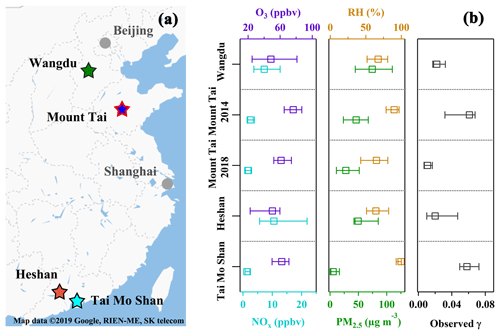Figure 1(a) The locations of the four field measurement sites (star markers) in China. (b) Comparisons of the concentrations of O3, NOx, PM2.5, and observed RH and ${\mathit{\gamma }}_{{\mathrm{N}}_{\mathrm{2}}{\mathrm{O}}_{\mathrm{5}}}$ during the five campaigns in China. Squares represent the median values and bars represent the interquartile ranges of the values in the five measurements. It should be noted that the high RH in Mt. Tai in 2014 and Tai Mo Shan campaigns were caused by frequent cloud or fog events, and the ${\mathit{\gamma }}_{{\mathrm{N}}_{\mathrm{2}}{\mathrm{O}}_{\mathrm{5}}}$ was determined only during noncloudy periods in these two campaigns.

In addition, the Community Multiscale Air Quality (CMAQ) model (v5.1) was employed to evaluate the uptake parameterization. Two simulations (default and revised) were conducted. In the default case, the N2O5 uptake and ClNO2 production were calculated based on the parameterization by Bertram and Thornton (2009). In the revised case, the new parameterization derived in this study was used. Other model configuration options were the same. The SAPRC07tic gas mechanism and AERO6i aerosol mechanism were used. The Weather Research and Forecasting (WRF) model (v4.0) was applied to generate the meteorological inputs for the CMAQ simulations. The anthropogenic emission inputs were generated based on the local Chinese emission inventory (Zhao et al., 2018) and the INTEX-B dataset for Asia (Zhang et al., 2009). The high-resolution chloride emission inventory for China from Fu et al. (2018) was also included. More details on the model configuration can be found in Fu et al. (2019). The simulation domain covers China with a resolution of 36 km×36 km (Fig. S1), based on a Lambert projection with two true latitudes of 25 and 40 N. The simulation period was from 1 to 31 December 2017, with 5 d before as a spin-up time.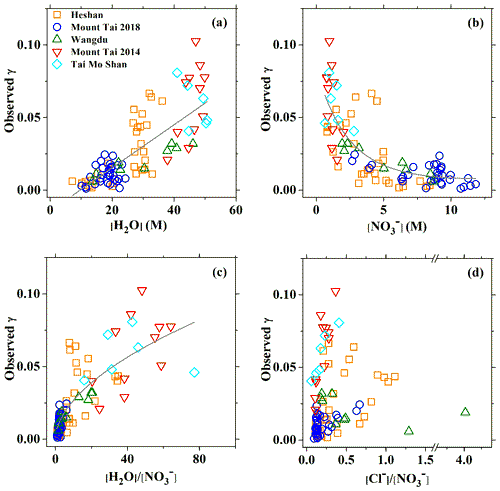Figure 2Relationship between the field-measured or derived N2O5 uptake coefficient ${\mathit{\gamma }}_{{\mathrm{N}}_{\mathrm{2}}{\mathrm{O}}_{\mathrm{5}}}$ and (a) aerosol water content, (b) particle nitrate, (c) H2O to ${\mathrm{NO}}_{\mathrm{3}}^{-}$ molarity ratio, and (d) Cl to ${\mathrm{NO}}_{\mathrm{3}}^{-}$ molarity ratio. Green triangles, red triangles, cyan diamond symbols, orange squares, and blue circles represent the results of Wangdu in 2014, Mount Tai in 2014, Tai Mo Shan in 2016, Heshan in 2017, and Mount Tai in 2018, respectively. The solid lines are linear or exponential regressions.

3 Results and discussion

## 3.1 Field-measured ${\mathit{\gamma }}_{{\mathrm{N}}_{\mathrm{2}}{\mathrm{O}}_{\mathrm{5}}}$ and influencing factors

During the field measurements at Heshan and Mt. Tai, the air was characterized as moderately polluted for O3 (43±22 ppbv at Heshan and 63±14 ppbv at Mt. Tai), NOx (14.0±11.5 ppbv at Heshan and 2.2±2.1 ppbv at Mt. Tai), and PM2.5 (66.7±41.9µg m−3 at Heshan and 33.7±26.7µg m−3 at Mt. Tai), as summarized in Table S2 and shown in Fig. 1b. ${\mathit{\gamma }}_{{\mathrm{N}}_{\mathrm{2}}{\mathrm{O}}_{\mathrm{5}}}$, which was directly measured using the aerosol flow tube, showed a large variation ranging from 0.002 to 0.067 with an average of 0.020±0.019 at Heshan and from 0.001 to 0.019 with an average of 0.011±0.005 at Mt. Tai. These values are within the range of 10−5 to > 0.1 derived from the ambient N2O5 concentrations around the world (e.g., Brown et al., 2006; Bertram et al., 2009b; Riedel et al., 2012; Morgan et al., 2015; Z. Wang et al., 2017; Tham et al., 2018; McDuffie et al., 2018a) but slightly lower than the previous results in the polluted regions in China (0.021 to 0.102) (Z. Wang et al., 2017; X. Wang et al., 2017; H. Wang et al., 2017). The field-measured ${\mathit{\gamma }}_{{\mathrm{N}}_{\mathrm{2}}{\mathrm{O}}_{\mathrm{5}}}$ and relevant pollutants at the two sites as well as those derived from three previous studies in China are summarized in Fig. 1b, covering diverse atmospheric conditions from moderately humid to humid conditions and from clean to polluted conditions.

Figure 2 shows the relationship between the field-measured ${\mathit{\gamma }}_{{\mathrm{N}}_{\mathrm{2}}{\mathrm{O}}_{\mathrm{5}}}$ and the aerosol composition during five campaigns at those four sites in China. It can be seen that the ${\mathit{\gamma }}_{{\mathrm{N}}_{\mathrm{2}}{\mathrm{O}}_{\mathrm{5}}}$ had a good positive correlation with the aerosol water content (r2=0.65) (Fig. 2a), suggesting a common controlling role of aerosol water in the reactivity of N2O5 in both northern and southern China. Although the positive correlation of ${\mathit{\gamma }}_{{\mathrm{N}}_{\mathrm{2}}{\mathrm{O}}_{\mathrm{5}}}$ with the humidity or aerosol water has been observed in the low range in previous laboratory studies, the ${\mathit{\gamma }}_{{\mathrm{N}}_{\mathrm{2}}{\mathrm{O}}_{\mathrm{5}}}$ reached plateaus at a value around 0.036 at RH > 50 % or [H2O] > 15 M (Hallquist et al., 2003; Thornton et al., 2003; Bertram and Thornton, 2009). In contrast, other laboratory studies also measured higher ${\mathit{\gamma }}_{{\mathrm{N}}_{\mathrm{2}}{\mathrm{O}}_{\mathrm{5}}}$ values on NH4HSO4 particles. For example, Mozurkewich and Calvert (1988) reported an upper limit of ${\mathit{\gamma }}_{{\mathrm{N}}_{\mathrm{2}}{\mathrm{O}}_{\mathrm{5}}}$ of 0.056 at RH = 55 % at 293 K, which increased to around 0.1 at 274 K. Kane et al. (2001) observed a strong RH-dependent ${\mathit{\gamma }}_{{\mathrm{N}}_{\mathrm{2}}{\mathrm{O}}_{\mathrm{5}}}$, increasing from 0.018 to 0.069 with RH from 56 % to 99 %, which is largely consistent with the field results in the present study. Moreover, several field measurements also observed ${\mathit{\gamma }}_{{\mathrm{N}}_{\mathrm{2}}{\mathrm{O}}_{\mathrm{5}}}$ values exceeding 0.04 at high RH or water molarity (e.g., Phillips et al., 2016; McDuffie et al., 2018a; H. Wang et al., 2017; Tham et al., 2018), and some of them also found the similar positive relationship between ${\mathit{\gamma }}_{{\mathrm{N}}_{\mathrm{2}}{\mathrm{O}}_{\mathrm{5}}}$ and water molarity (McDuffie et al., 2018a). Although uncertainties may exist in the calculation of aerosol surface and uptake coefficient at high ambient RH conditions, our results with a consistently increasing trend of ${\mathit{\gamma }}_{{\mathrm{N}}_{\mathrm{2}}{\mathrm{O}}_{\mathrm{5}}}$ with [H2O] from below 10 M up to 50 M suggest that the aerosol water content strongly affects the activity of N2O5 uptake, and that N2O5 hydrolysis is always limited by aerosol water content under all the encountered ambient conditions. Since limited measurement data of ${\mathit{\gamma }}_{{\mathrm{N}}_{\mathrm{2}}{\mathrm{O}}_{\mathrm{5}}}$ from laboratory and field studies are available at the RH > 80 % condition, it is unclear what exact mechanism or process (e.g., a phase change different from laboratory-made particles or acidity involved) promotes more effective uptake on ambient aerosols at higher aerosol water content conditions. Therefore, more detailed investigations of N2O5 uptake on nanosized water or aerosol droplets in the real (or close to real) ambient conditions are clearly warranted. For nitrate, a clear suppression effect can be found at the Chinese sites (Fig. 2b), which is similar to most of the previous field and laboratory studies. The decrease of ${\mathit{\gamma }}_{{\mathrm{N}}_{\mathrm{2}}{\mathrm{O}}_{\mathrm{5}}}$ with increasing nitrate concentration seems to be better captured by an “exponential-decay” curve, with almost linear suppression for [${\mathrm{NO}}_{\mathrm{3}}^{-}$] below 5 M. The observed ${\mathit{\gamma }}_{{\mathrm{N}}_{\mathrm{2}}{\mathrm{O}}_{\mathrm{5}}}$ under high nitrate condition (> 5 M) was generally below 0.025 and became nitrate independent as the nitrate levels further increased.

The ${\mathit{\gamma }}_{{\mathrm{N}}_{\mathrm{2}}{\mathrm{O}}_{\mathrm{5}}}$ variation was affected by the additive effects from both [${\mathrm{NO}}_{\mathrm{3}}^{-}$] and [H2O], which could not be easily isolated because of their competing reactions with the reactive intermediate ${\mathrm{H}}_{\mathrm{2}}{\mathrm{ONO}}_{\mathrm{2}}^{+}$. This is further supported by the positive dependence of ${\mathit{\gamma }}_{{\mathrm{N}}_{\mathrm{2}}{\mathrm{O}}_{\mathrm{5}}}$ on the molar ratio of $\left[{\mathrm{H}}_{\mathrm{2}}\mathrm{O}\right]/\left[{\mathrm{NO}}_{\mathrm{3}}^{-}\right]$ (Fig. 2c). Different from the previously reported plateauing of ${\mathit{\gamma }}_{{\mathrm{N}}_{\mathrm{2}}{\mathrm{O}}_{\mathrm{5}}}$ with increasing $\left[{\mathrm{H}}_{\mathrm{2}}\mathrm{O}\right]/\left[{\mathrm{NO}}_{\mathrm{3}}^{-}\right]$ ratio in laboratory studies (Hallquist et al., 2003; Bertram and Thornton, 2009), no decrease in ${\mathit{\gamma }}_{{\mathrm{N}}_{\mathrm{2}}{\mathrm{O}}_{\mathrm{5}}}$ suppression was found in the present study for $\left[{\mathrm{H}}_{\mathrm{2}}\mathrm{O}\right]/\left[{\mathrm{NO}}_{\mathrm{3}}^{-}\right]$ ratio of up to 60. The more scattered data at higher $\left[{\mathrm{H}}_{\mathrm{2}}\mathrm{O}\right]/\left[{\mathrm{NO}}_{\mathrm{3}}^{-}\right]$ ranges imply that the variation of ${\mathit{\gamma }}_{{\mathrm{N}}_{\mathrm{2}}{\mathrm{O}}_{\mathrm{5}}}$ becomes more sensitive to other factors in the diluted aqueous aerosols. Although the ${\mathit{\gamma }}_{{\mathrm{N}}_{\mathrm{2}}{\mathrm{O}}_{\mathrm{5}}}$ measured at two mountain sites showed a positive relationship with $\left[{\mathrm{Cl}}^{-}\right]/\left[{\mathrm{NO}}_{\mathrm{3}}^{-}\right]$, the overall results from five sites did not exhibit an obvious pattern (Fig. 2d). These results suggest that chloride concentration may not play a critical role in ${\mathit{\gamma }}_{{\mathrm{N}}_{\mathrm{2}}{\mathrm{O}}_{\mathrm{5}}}$ during our observations as laboratory studies have observed (Bertram and Thornton, 2009), possibly due to the complex effect of aerosol mixing state. Though the measured ${\mathit{\gamma }}_{{\mathrm{N}}_{\mathrm{2}}{\mathrm{O}}_{\mathrm{5}}}$ exhibited a nonlinear relationship and complex dependence on different factors at a single site, the general consistent patterns at different sites in this study suggests the feasibility of a common parameterization representing the N2O5 uptake in these regions.

Table 1Statistical summary and comparison of the observed parameters (N2O5 uptake coefficient, ${\mathit{\gamma }}_{{\mathrm{N}}_{\mathrm{2}}{\mathrm{O}}_{\mathrm{5}}}$; ClNO2 yield, ${\mathrm{\Phi }}_{{\mathrm{ClNO}}_{\mathrm{2}}}$) with values predicted from different parameterizations.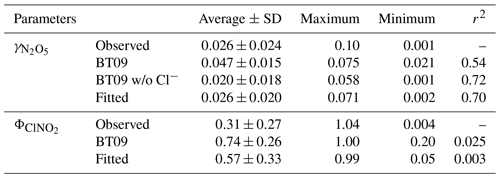## 3.2 Comparison to parameterizations

Current regional air quality models such as WRF-Chem and CMAQ mainly use the ${\mathit{\gamma }}_{{\mathrm{N}}_{\mathrm{2}}{\mathrm{O}}_{\mathrm{5}}}$ parameterization recommended by Bertram and Thornton (2009) (hereafter referred to as BT09), which links ${\mathit{\gamma }}_{{\mathrm{N}}_{\mathrm{2}}{\mathrm{O}}_{\mathrm{5}}}$ to aerosol water content, nitrate, and chloride as well as the aerosol size and ambient temperature. The BT09 parameterization based on the abovementioned reaction mechanism (Reactions R1–R5) was expressed as follows:

$\begin{array}{}\text{(2)}& \begin{array}{rl}{\mathit{\gamma }}_{{\mathrm{N}}_{\mathrm{2}}{\mathrm{O}}_{\mathrm{5}}}=& \frac{\mathrm{4}}{c}\frac{{V}_{\text{a}}}{{S}_{\text{a}}}{K}_{\text{H}}{{k}^{\prime }}_{\text{2f}}\\ & \left(\mathrm{1}-\frac{\mathrm{1}}{\left(\frac{{k}_{\mathrm{3}}\left[{\mathrm{H}}_{\mathrm{2}}\mathrm{O}\right]}{{k}_{\text{2b}}\left[{\mathrm{NO}}_{\mathrm{3}}^{-}\right]}\right)+\mathrm{1}+\left(\frac{{k}_{\mathrm{4}}\left[{\mathrm{Cl}}^{-}\right]}{{k}_{\text{2b}}\left[{\mathrm{NO}}_{\mathrm{3}}^{-}\right]}\right)}\right)\end{array},\text{(3)}& {{k}^{\prime }}_{\text{2f}}=\mathit{\beta }-\mathit{\beta }{e}^{\left(-\mathit{\delta }\left[{\mathrm{H}}_{\mathrm{2}}\mathrm{O}\right]\right)},\end{array}$

where VaSa is the measured aerosol volume to surface area ratio, ranging from $\mathrm{3.30}×{\mathrm{10}}^{-\mathrm{8}}$ to $\mathrm{9.29}×{\mathrm{10}}^{-\mathrm{8}}$ m in the five campaigns; KH is Henry's law coefficient, taken as 51 (Bertram and Thornton, 2009; Fried et al., 1994); $\mathit{\beta }=\mathrm{1.15}×{\mathrm{10}}^{\mathrm{6}}$; $\mathit{\delta }=-\mathrm{0.13}$. The parameters ${k}_{\mathrm{3}}/{k}_{\text{2b}}\left(=\mathrm{0.06}\right)$ and ${k}_{\mathrm{4}}/{k}_{\text{2b}}\left(=\mathrm{29}\right)$ represent the relative rates of competing reactions of intermediate ${\mathrm{H}}_{\mathrm{2}}{\mathrm{ONO}}_{\mathrm{2}}^{+}\left(\mathrm{aq}\right)$ with H2O (Reaction R3) and Cl (Reaction R4) over ${\mathrm{NO}}_{\mathrm{3}}^{-}$ (Reaction R2), respectively. [H2O], [${\mathrm{NO}}_{\mathrm{3}}^{-}$], and [Cl] are the aerosol water content, aerosol nitrate, and chloride molarity, respectively, calculated by the E-AIM model with the measured ionic compositions of PM2.5 and RH (http://www.aim.env.uea.ac.uk/aim/aim.php, last access: 8 April 2020) (Wexler and Clegg, 2002).

We calculate the ${\mathit{\gamma }}_{{\mathrm{N}}_{\mathrm{2}}{\mathrm{O}}_{\mathrm{5}}}$ values from BT09 with the measured aerosol composition at the five sites. The parameterized ${\mathit{\gamma }}_{{\mathrm{N}}_{\mathrm{2}}{\mathrm{O}}_{\mathrm{5}}}$ ranged from 0.021 to 0.075, with an average of 0.047±0.015, which overestimates the observed values by a factor of 1.8. When the chloride effect was excluded, the parameterized ${\mathit{\gamma }}_{{\mathrm{N}}_{\mathrm{2}}{\mathrm{O}}_{\mathrm{5}}}$ mean value decreased to 0.020±0.018, which was better correlated with but underestimated (by 30 %) the measurements (Table 1). Figure 3 compares the observation-derived and parameterized ${\mathit{\gamma }}_{{\mathrm{N}}_{\mathrm{2}}{\mathrm{O}}_{\mathrm{5}}}$ at five sites in China and in different parts of the world. The BT09 parameterization (blue markers in Fig. 3) generally overestimates the observed ${\mathit{\gamma }}_{{\mathrm{N}}_{\mathrm{2}}{\mathrm{O}}_{\mathrm{5}}}$ values in the range of 0.001 to 0.03, but it is closer (within a factor of 1.5) to the observed value for ${\mathit{\gamma }}_{{\mathrm{N}}_{\mathrm{2}}{\mathrm{O}}_{\mathrm{5}}}$ above 0.03 in Germany (Phillips et al., 2016) and Mt. Tai (Z. Wang et al., 2017). The BT09 parameterization excluding chloride effects (orange markers) gives much better agreement, with more values located in the range within a factor of 2, though the ${\mathit{\gamma }}_{{\mathrm{N}}_{\mathrm{2}}{\mathrm{O}}_{\mathrm{5}}}$ was still overpredicted in most of the studies in North America (Bertram et al., 2009b; Riedel et al., 2012; McDuffie et al., 2018a) except for Boulder (Wagner et al., 2013). The improvement indicates that the efficiency of chloride in competing for the ${\mathrm{H}}_{\mathrm{2}}{\mathrm{ONO}}_{\mathrm{2}}^{+}$ intermediate and the effects on N2O5 uptake on ambient aerosols might be overestimated, possibly due to the existence of other nucleophiles competing with Cl (McDuffie et al., 2018b; Staudt et al., 2019) or different mixing states of particle and nonuniform distribution of available chlorine in the aerosols.

Organic matter or coatings on the aerosols can suppress the uptake of N2O5 (Thornton and Abbatt, 2005; McNeill et al., 2006; Park et al., 2007), and previous studies have attempted to account for this effect by treating organics as a coating on the inorganic core (Anttila et al., 2006; Riemer et al., 2009). However, significant underpredictions were found from the parameterization of BT09 combined with the organic effect (Morgan et al., 2015; McDuffie et al., 2018a; Tham et al., 2018) (green and red markers in Fig. 3). One reason could be that the parameterization does not differentiate the water-soluble organic fractions and simplifies the morphology, and phase state, which leads to the underestimation of the solubility and/or diffusivity of N2O5 in the organics. The complex effects of organic matter on N2O5 uptake remain poorly quantified (McDuffie et al., 2018a), and the prediction of composition, morphology and phase state of the organic fractions are still difficult in current air quality models. Therefore, we do not consider the organic effect in deriving a new parameterization in the next section.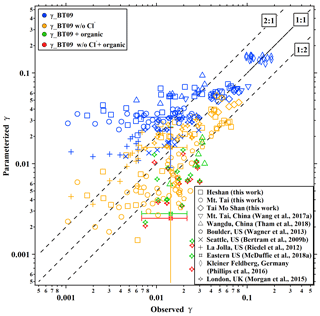Figure 3Summary of the comparisons of field-measured or derived ${\mathit{\gamma }}_{{\mathrm{N}}_{\mathrm{2}}{\mathrm{O}}_{\mathrm{5}}}$ and values estimated from parameterizations from the literature. Blue, orange, green, and red markers represent the results calculated from parameterizations of original BT09, BT09 excluding chloride effect, BT09 plus organic effect, and BT09 excluding chloride but with organic effect, respectively.

## 3.3 Observation-based empirical parameterization of ${\mathit{\gamma }}_{{\mathrm{N}}_{\mathrm{2}}{\mathrm{O}}_{\mathrm{5}}}$

Based on the above discussion and comparison, we attempt to derive a new empirical parameterization of ${\mathit{\gamma }}_{{\mathrm{N}}_{\mathrm{2}}{\mathrm{O}}_{\mathrm{5}}}$ following the BT09 framework (Eq. 2) and using the measurement data from five field campaigns in China. The variables in the parameterization (i.e., reaction rates) were fitted with multiple regressions to obtain the best representation of observations in China. The derived empirical parameterization of ${\mathit{\gamma }}_{{\mathrm{N}}_{\mathrm{2}}{\mathrm{O}}_{\mathrm{5}}}$ is shown as Eq. (4) and the fitted ${\mathit{\gamma }}_{{\mathrm{N}}_{\mathrm{2}}{\mathrm{O}}_{\mathrm{5}}}$ values are summarized in Table 1.

$\begin{array}{}\text{(4)}& \begin{array}{rl}{\mathit{\gamma }}_{{\mathrm{N}}_{\mathrm{2}}{\mathrm{O}}_{\mathrm{5}}}=& \frac{\mathrm{4}}{c}\frac{{V}_{\text{a}}}{{S}_{\text{a}}}{K}_{\text{H}}×\mathrm{3.0}×{\mathrm{10}}^{\mathrm{4}}×\left[{\mathrm{H}}_{\mathrm{2}}\mathrm{O}\right]\\ & \left(\mathrm{1}-\frac{\mathrm{1}}{\left(\mathrm{0.033}×\frac{\left[{\mathrm{H}}_{\mathrm{2}}\mathrm{O}\right]}{\left[{\mathrm{NO}}_{\mathrm{3}}^{-}\right]}\right)+\mathrm{1}+\left(\mathrm{3.4}×\frac{\left[{\mathrm{Cl}}^{-}\right]}{\left[{\mathrm{NO}}_{\mathrm{3}}^{-}\right]}\right)}\right)\end{array}\end{array}$

In view of the linear dependence of ${\mathit{\gamma }}_{{\mathrm{N}}_{\mathrm{2}}{\mathrm{O}}_{\mathrm{5}}}$ on the aerosol water content in this study and reaction mechanism (Bertram and Thornton, 2009), the second-order reaction rate coefficient with water (refer to ${{k}^{\prime }}_{\text{2f}}$ in Eqs. 2 and 3) was fitted as a linear function of [H2O], as $\left(\mathrm{3.0}±\mathrm{0.4}\right)×{\mathrm{10}}^{\mathrm{4}}×\left[{\mathrm{H}}_{\mathrm{2}}\mathrm{O}\right]$. This value is in reasonable agreement with the values of (2.7–3.8) ×104, $\sim \mathrm{3.9}×{\mathrm{10}}^{\mathrm{4}}$, and 2.6×104 M−1 s−1 determined from ammonium bisulfate, ammonium sulfate (Gaston and Thornton, 2016), and aqueous organic acid particles (Thornton et al., 2003), respectively. Compared to original BT09 (Eq. 3), the newly fitted ${{k}^{\prime }}_{\text{2f}}$ is smaller for [H2O] < 38 M, but it become higher with the increasing of aerosol water content (Fig. S2). Different dimensionless KH values have been used in previous studies, e.g.,  50 (e.g., Hallquist et al., 2003; Bertram and Thornton, 2009) or  120 (e.g., Gaston et al., 2014; Gaston and Thornton, 2016; Griffiths et al., 2009), which correspond to a Henry's law constant of 2 or 5 M atm−1 at 298 K. As ${\mathit{\gamma }}_{{\mathrm{N}}_{\mathrm{2}}{\mathrm{O}}_{\mathrm{5}}}$ in the parameterization is linearly dependent on the KH, an increase of KH value would proportionally increase the ${\mathit{\gamma }}_{{\mathrm{N}}_{\mathrm{2}}{\mathrm{O}}_{\mathrm{5}}}$ value, but it cannot account for the large variability of measured ${\mathit{\gamma }}_{{\mathrm{N}}_{\mathrm{2}}{\mathrm{O}}_{\mathrm{5}}}$ values. Given the lack of an explicit function of effective Henry's law constant for N2O5 to include the different processes (e.g., “salting-in” effect and surface processes), we use the value of 51 suggested by Bertram and Thornton (2009) and enclose those effects from the aerosol composition in the last “chemical” term. The derived empirical ratios in the last chemical term not only represent the competing ratio of these reactions but also include other unspecified effects or processes (organic coating, mixing state, other nucleophiles reactions, etc.). The fitted relative rates of competing reactions, i.e., k3k2b and k4k2b, were 0.033±0.017 and 3.4±1.4, respectively, which are smaller than the original BT09 parameters by factors of 1.8 and 8.5, respectively. The smaller ratios of the reaction rates indicate a smaller enhancement effect of chloride or a larger suppression effect by nitrate, which is consistent with the abovementioned relationship of ${\mathit{\gamma }}_{{\mathrm{N}}_{\mathrm{2}}{\mathrm{O}}_{\mathrm{5}}}$ with the aerosol composition. Other suppression effects such as organic coating and mixing state that were not specified in the parameterization also may contribute to, and are reflected in, the smaller fitted values. As compared in Fig. 4 and Table 1, the new empirical parameterization can better reproduce the average values and the large variability of the observed ${\mathit{\gamma }}_{{\mathrm{N}}_{\mathrm{2}}{\mathrm{O}}_{\mathrm{5}}}$ than the original BT09 both with and without considering Cl effects.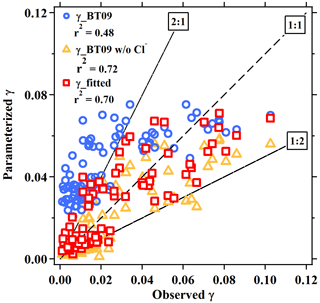Figure 4Comparison of the field-measured or derived ${\mathit{\gamma }}_{{\mathrm{N}}_{\mathrm{2}}{\mathrm{O}}_{\mathrm{5}}}$ with the values estimated from parameterizations for the five sites in the present study. The dashed line represents the 1 : 1 line. Blue circles, orange triangles, and red squares are results estimated by BT09 parameterization, BT09 excluding chloride effect and the derived observation-based empirical parameterization, respectively.

Table 2Statistical summary and comparison of the observed species (nitrate and NO2 concentrations) with values predicted from different parameterization. NMB represents the normalized mean bias.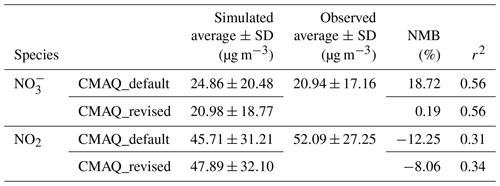As suggested by the previous studies, the production yield of ClNO2 (${\mathrm{\Phi }}_{{\mathrm{ClNO}}_{\mathrm{2}}}$) from N2O5 uptake is also a function of competing reactions of H2O and Cl content in aerosols, and they can be estimated from ${\mathrm{\Phi }}_{{\mathrm{ClNO}}_{\mathrm{2}}}=\mathrm{1}/\left(\mathrm{1}+{k}_{\mathrm{3}}/{k}_{\mathrm{4}}×\left[{\mathrm{H}}_{\mathrm{2}}\mathrm{O}\right]/\left[{\mathrm{Cl}}^{-}\right]\right)$ (Bertram and Thornton, 2009). Based on the abovementioned fitting results for ${\mathit{\gamma }}_{{\mathrm{N}}_{\mathrm{2}}{\mathrm{O}}_{\mathrm{5}}}$, k4k3 is determined to be 105±37 for the five sites, which is smaller than the values of 450±100 (Roberts et al., 2009), 483±175 (Bertram and Thornton, 2009) and 836±32 (Behnke et al., 1997) derived from laboratory experiments and used in previous parameterizations. As compared in Fig. S3 and Table 1, although the newly fitted values improve the estimated ClNO2 yield comparing to the original BT09 (with k4k3 of 483), overestimation remains, and the large variability of observed ${\mathrm{\Phi }}_{{\mathrm{ClNO}}_{\mathrm{2}}}$ in different campaigns still cannot be well captured. As shown in Fig. 5, the new fits can better catch the ${\mathrm{\Phi }}_{{\mathrm{ClNO}}_{\mathrm{2}}}$ trend at $\left[{\mathrm{H}}_{\mathrm{2}}\mathrm{O}\right]/\left[{\mathrm{Cl}}^{-}\right]>\mathrm{750}$, but a discrepancy is still obvious at $\left[{\mathrm{H}}_{\mathrm{2}}\mathrm{O}\right]/\left[{\mathrm{Cl}}^{-}\right]<\mathrm{750}$. The discrepancy could be due to aqueous-phase competition reactions of intermediate ${\mathrm{H}}_{\mathrm{2}}{\mathrm{ONO}}_{\mathrm{2}}^{+}$ with other compounds (e.g., phenol) (Heal et al., 2007) and ClNO2 loss or reaction mechanisms (e.g., reaction with Cl to form Cl2) (Roberts et al., 2008, 2009). A recent laboratory study (Staudt et al., 2019) has reported that sulfate and acetate can suppress ${\mathrm{\Phi }}_{{\mathrm{ClNO}}_{\mathrm{2}}}$ for Cl-containing solutions, but such a sulfate suppression effect was not observed in our results. Further studies are needed to identify and quantify these effects for better parameterizing the heterogeneous ClNO2 production. Nonetheless, the revised k3k4 from fitting the field data has improved the estimates of ${\mathrm{\Phi }}_{{\mathrm{ClNO}}_{\mathrm{2}}}$ at our study sites.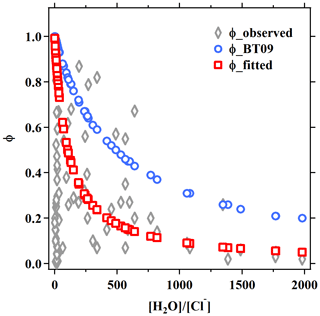Figure 5Relationship between the ClNO2 yield, ${\mathrm{\Phi }}_{{\mathrm{ClNO}}_{\mathrm{2}}}$, and the molarity ratio of H2O to Cl. Gray diamond symbols, blue circles, and red squares represent the observed ${\mathrm{\Phi }}_{{\mathrm{ClNO}}_{\mathrm{2}}}$, values from BT09 parameterization, and those fitted from the empirical parameters derived in the present study, respectively.

## 3.4 Evaluation of the empirical parameterization

To further evaluate the representativeness and validity of the newly fitted empirical parameterization of ${\mathit{\gamma }}_{{\mathrm{N}}_{\mathrm{2}}{\mathrm{O}}_{\mathrm{5}}}$ in predicting N2O5 heterogeneous process in air quality models, simulation tests were performed with the WRF-CMAQ model. The simulations were conducted with the incorporation of newly fitted and original BT09 parameterizations, respectively. The simulated concentrations of NO2 and ${\mathrm{NO}}_{\mathrm{3}}^{-}$, as the key precursor and a product of the N2O5 uptake, were compared with the observed daily ${\mathrm{NO}}_{\mathrm{3}}^{-}$ concentrations at 28 sites and hourly NO2 concentrations at 472 sites in the North China Plain during December of 2017. As summarized in Table 2 and shown in Fig. S4, the simulation with original BT09 parameterization overestimated the regionally averaged ${\mathrm{NO}}_{\mathrm{3}}^{-}$ concentrations by 18.7 % compared to the observations, whereas the new parameterization gave more consistent results with the observations (20.98±18.77µg m−3 vs. 20.94±17.16µg m−3), reducing the normalized mean bias (NMB) of simulated ${\mathrm{NO}}_{\mathrm{3}}^{-}$ concentration from 18.72 % to 0.19 %. The simulated NO2 concentrations were also in better agreement with the observations, with the NMB changing from −12.25 % to −8.06 %. In addition to NO2 and ${\mathrm{NO}}_{\mathrm{3}}^{-}$, we also compared the simulated N2O5 concentrations for December 2017 with those observed in the wintertime at various locations in China, including two in the North China Plain (Beijing and Wangdu in Hebei Province) and two in southern China (Tai Mo Shan and Heshan). As shown in Fig. 6, with the new parameterization, the WRF-CMAQ model can better simulate the average concentration and variation range of N2O5 at these locations. Overall, the new parameterization has significantly reduced the discrepancies between the modeled and observed concentrations of NO2, N2O5, and ${\mathrm{NO}}_{\mathrm{3}}^{-}$ at our study sites and periods in both northern and southern China. More tests of this empirical parameterization are warranted for other locations and seasons in China and other parts of the world.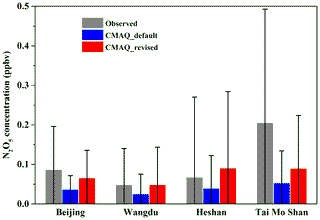Figure 6Comparison of simulated N2O5 concentrations by the CMAQ model for December 2017 with the wintertime observation results from four sites in China. The field observations were conducted in Wangdu (Hebei Province) in December 2017, Beijing in January 2018, Heshan (Guangdong Province) in January 2017, and Tai Mo Shan (Hong Kong) in November 2013. The columns and error bars represent the average value and standard deviation, respectively.

4 Conclusion

Nitrate is becoming the predominant component of PM2.5 during severe haze events in China in recent years (Zhang et al., 2015; Li et al., 2018), and ground-level ozone pollution in urban areas is also worsening (T. Wang et al., 2017). Despite extensive research, current air quality models still have difficulties in accurately simulating the N2O5 uptake on aerosols, which limits their ability to predict the lifetime and fate of NOx and therefore the production of aerosol nitrate and ozone. Based on the measurements from five field campaigns at four sites across China with different atmospheric conditions, our study examined the factors influencing N2O5 uptake processes and derived an observation-based empirical parameterization of N2O5 uptake. While further research is still needed on the additional factors affecting ${\mathit{\gamma }}_{{\mathrm{N}}_{\mathrm{2}}{\mathrm{O}}_{\mathrm{5}}}$ and ${\mathrm{\Phi }}_{{\mathrm{ClNO}}_{\mathrm{2}}}$, the empirical parameterization derived here can be used in air quality models to improve the prediction of PM2.5 and photochemical pollution in China and similar polluted regions of the world.

Data availability

The data used in this study are available upon request from Zhe Wang (z.wang@ust.hk) and Tao Wang (cetwang@polyu.edu.hk).

Supplement

Author contributions

TW and ZW designed the study. WW, CY, and ZW designed the aerosol flow tube, and CY carried out the aerosol flow-tube measurements. MX, TC, PZ, HL, YS, YZ, and DY conducted the field measurement of relevant species and data analysis. XF performed the model simulation. CY, ZW, and TW wrote the article, with discussions and comments from all co-authors.

Competing interests

The authors declare that they have no conflict of interest.

Special issue statement

Acknowledgements

The authors would like to acknowledge the Hong Kong Environmental Protection Department for their help with access to the TMS AQM station. The authors greatly acknowledge the editor and anonymous reviewers for their constructive suggestions.

Financial support

This research has been supported by the National Natural Science Foundation of China (grant nos. 91544213 and 91844301) and the Research Grants Council of Hong Kong Special Administrative Region (grant nos. T24/504/17, C5022-14G, and 15265516).

Review statement

This paper was edited by Jingkun Jiang and reviewed by two anonymous referees.

References

Anttila, T., Kiendler-Scharr, A., Tillmann, R., and Mentel, T. F.: On the Reactive Uptake of Gaseous Compounds by Organic-Coated Aqueous Aerosols: Theoretical Analysis and Application to the Heterogeneous Hydrolysis of N2O5, J. Phys. Chem. A, 110, 10435–10443, https://doi.org/10.1021/jp062403c, 2006.

Atkinson, R. and Arey, J.: Gas-phase tropospheric chemistry of biogenic volatile organic compounds: a review, Atmos. Environ., 37, 197–219, https://doi.org/10.1016/s1352-2310(03)00391-1, 2003.

Behnke, W., George, C., Scheer, V., and Zetzsch, C.: Production and decay of ClNO2 from the reaction of gaseous N2O5 with NaCl solution: Bulk and aerosol experiments, J. Geophys. Res.-Atmos., 102, 3795–3804, https://doi.org/10.1029/96jd03057, 1997.

Bertram, T. H. and Thornton, J. A.: Toward a general parameterization of N2O5 reactivity on aqueous particles: the competing effects of particle liquid water, nitrate and chloride, Atmos. Chem. Phys., 9, 8351–8363, https://doi.org/10.5194/acp-9-8351-2009, 2009.

Bertram, T. H., Thornton, J. A., and Riedel, T. P.: An experimental technique for the direct measurement of N2O5 reactivity on ambient particles, Atmos. Meas. Tech., 2, 231–242, https://doi.org/10.5194/amt-2-231-2009, 2009a.

Bertram, T. H., Thornton, J. A., Riedel, T. P., Middlebrook, A. M., Bahreini, R., Bates, T. S., Quinn, P. K., and Coffman, D. J.: Direct observations of N2O5 reactivity on ambient aerosol particles, Geophys. Res. Lett., 36, L19803, https://doi.org/10.1029/2009gl040248, 2009b.

Brown, S., Ryerson, T., Wollny, A., Brock, C., Peltier, R., Sullivan, A., Weber, R., Dube, W., Trainer, M., and Meagher, J. F.: Variability in nocturnal nitrogen oxide processing and its role in regional air quality, Science, 311, 67–70, 2006.

Brown, S. S., Dubé, W. P., Fuchs, H., Ryerson, T. B., Wollny, A. G., Brock, C. A., Bahreini, R., Middlebrook, A. M., Neuman, J. A., and Atlas, E.: Reactive uptake coefficients for N2O5 determined from aircraft measurements during the Second Texas Air Quality Study: Comparison to current model parameterizations, J. Geophys. Res.-Atmos., 114, D00F10, https://doi.org/10.1029/2008JD011679, 2009.

Brown, S. S., Dubé, W. P., Fuchs, H., Ryerson, T. B., Wollny, A. G., Brock, C. A., Bahreini, R., Middlebrook, A. M., Neuman, J. A., Atlas, E., Roberts, J. M., Osthoff, H. D., Trainer, M., Fehsenfeld, F. C., and Ravishankara, A. R.: Reactive uptake coefficients for N2O5 determined from aircraft measurements during the Second Texas Air Quality Study: Comparison to current model parameterizations, J. Geophys. Res., 114, D00F10, https://doi.org/10.1029/2008jd011679, 2009.

Brown, S. S., Dubé, W. P., Tham, Y. J., Zha, Q., Xue, L., Poon, S., Wang, Z., Blake, D. R., Tsui, W., and Parrish, D. D.: Nighttime chemistry at a high altitude site above Hong Kong, J. Geophys. Res.-Atmos., 121, 2457–2475, 2016.

Cosman, L. M., Knopf, D. A., and Bertram, A. K.: N2O5 reactive uptake on aqueous sulfuric acid solutions coated with branched and straight-chain insoluble organic surfactants, J. Phys. Chem. A, 112, 2386–2396, 2008.

Davis, J. M., Bhave, P. V., and Foley, K. M.: Parameterization of N2O5 reaction probabilities on the surface of particles containing ammonium, sulfate, and nitrate, Atmos. Chem. Phys., 8, 5295–5311, https://doi.org/10.5194/acp-8-5295-2008, 2008.

Evans, M. and Jacob, D. J.: Impact of new laboratory studies of N2O5 hydrolysis on global model budgets of tropospheric nitrogen oxides, ozone, and OH, Geophys. Res. Lett., 32, L09813, https://doi.org/10.1029/2005GL022469, 2005.

Finlayson-Pitts, B. J., Ezell, M. J., and Pitts, J. N.: Formation of chemically active chlorine compounds by reactions of atmospheric NaCl particles with gaseous N2O5 and ClONO2, Nature, 337, 241–244, 1989.

Fried, A., Henry, B. E., Calvert, J. G., and Mozurkewich, M.: The reaction probability of N2O5 with sulfuric-acid aerosols at stratospheric temperatures and compositions, J. Geophys. Res., 99, 3517–3532, 1994.

Fu, X., Wang, T., Wang, S., Zhang, L., Cai, S., Xing, J., and Hao, J.: Anthropogenic emissions of hydrogen chloride and fine particulate chloride in China, Environ. Sci. Technol., 52, 1644–1654, 2018.

Fu, X., Wang, T., Zhang, L., Li, Q., Wang, Z., Xia, M., Yun, H., Wang, W., Yu, C., Yue, D., Zhou, Y., Zheng, J., and Han, R.: The significant contribution of HONO to secondary pollutants during a severe winter pollution event in southern China, Atmos. Chem. Phys., 19, 1–14, https://doi.org/10.5194/acp-19-1-2019, 2019.

Gaston, C. J. and Thornton, J. A.: Reacto-diffusive length of N2O5 in aqueous sulfate-and chloride-containing aerosol particles, J. Phys. Chem. A, 120, 1039–1045, 2016.

Gaston, C. J., Thornton, J. A., and Ng, N. L.: Reactive uptake of N2O5 to internally mixed inorganic and organic particles: the role of organic carbon oxidation state and inferred organic phase separations, Atmos. Chem. Phys., 14, 5693–5707, https://doi.org/10.5194/acp-14-5693-2014, 2014.

Griffiths, P. T., Badger, C. L., Cox, R. A., Folkers, M., Henk, H. H., and Mentel, T. F.: Reactive uptake of N2O5 by aerosols containing dicarboxylic acids. Effect of particle phase, composition, and nitrate content, J. Phys. Chem. A, 113, 5082–5090, 2009.

Hallquist, M., Stewart, D. J., Stephenson, S. K., and Anthony Cox, R.: Hydrolysis of N2O5 on sub-micron sulfate aerosols, Phys. Chem. Chem. Phys., 5, 3453–3463, https://doi.org/10.1039/b301827j, 2003.

Heal, M. R., Harrison, M. A. J., and Neil Cape, J.: Aqueous-phase nitration of phenol by N2O5 and ClNO2, Atmos. Environ., 41, 3515–3520, https://doi.org/10.1016/j.atmosenv.2007.02.003, 2007.

Hennig, T., Massling, A., Brechtel, F. J., and Wiedensohler, A.: A Tandem DMA for highly temperature-stabilized hygroscopic particle growth measurements between 90 % and 98 % relative humidity, J. Aerosol Sci., 36, 1210–1223, https://doi.org/10.1016/j.jaerosci.2005.01.005, 2005.

Jiang, J., Kim, C., Wang, X., Stolzenburg, M. R., Kaufman, S. L., Qi, C., Sem, G. J., Sakurai, H., Hama, N., and McMurry, P. H.: Aerosol Charge Fractions Downstream of Six Bipolar Chargers: Effects of Ion Source, Source Activity, and Flowrate, Aerosol Sci. Technol., 48, 1207–1216, 2014.

Kane, S. M., Caloz, F., and Leu, M.-T.: Heterogeneous uptake of gaseous N2O5 by (NH4)2SO4, NH4HSO4, and H2SO4 aerosols, J. Phys. Chem. A, 105, 6465–6470, 2001.

Kuang, C.: TSI Model 3936 scanning mobility particle spectrometer instrument handbook, ARM Climate Research Facility, Washington, DC, USA, 2016.

Li, H., Zhang, Q., Zheng, B., Chen, C., Wu, N., Guo, H., Zhang, Y., Zheng, Y., Li, X., and He, K.: Nitrate-driven urban haze pollution during summertime over the North China Plain, Atmos. Chem. Phys., 18, 5293–5306, https://doi.org/10.5194/acp-18-5293-2018, 2018.

Li, Q., Zhang, L., Wang, T., Tham, Y. J., Ahmadov, R., Xue, L., Zhang, Q., and Zheng, J.: Impacts of heterogeneous uptake of dinitrogen pentoxide and chlorine activation on ozone and reactive nitrogen partitioning: improvement and application of the WRF-Chem model in southern China, Atmos. Chem. Phys., 16, 14875–14890, https://doi.org/10.5194/acp-16-14875-2016, 2016.

Liu, H. J., Zhao, C. S., Nekat, B., Ma, N., Wiedensohler, A., van Pinxteren, D., Spindler, G., Müller, K., and Herrmann, H.: Aerosol hygroscopicity derived from size-segregated chemical composition and its parameterization in the North China Plain, Atmos. Chem. Phys., 14, 2525–2539, https://doi.org/10.5194/acp-14-2525-2014, 2014.

McDuffie, E. E., Fibiger, D. L., Dubé, W. P., Lopez-Hilfiker, F., Lee, B. H., Thornton, J. A., Shah, V., Jaeglé, L., Guo, H., and Weber, R. J.: Heterogeneous N2O5 uptake during winter: Aircraft measurements during the 2015 WINTER campaign and critical evaluation of current parameterizations, J. Geophys. Res.-Atmos., 123, 4345–4372, 2018a.

McDuffie, E. E., Fibiger, D. L., Dubé, W. P., Lopez Hilfiker, F., Lee, B. H., Jaeglé, L., Guo, H., Weber, R. J., Reeves, J. M., and Weinheimer, A. J.: ClNO2 yields from aircraft measurements during the 2015 WINTER campaign and critical evaluation of the current parameterization, J. Geophys. Res.-Atmos., 123, 12994–13015, 2018b.

McNeill, V. F., Patterson, J., Wolfe, G. M., and Thornton, J. A.: The effect of varying levels of surfactant on the reactive uptake of N2O5 to aqueous aerosol, Atmos. Chem. Phys., 6, 1635–1644, https://doi.org/10.5194/acp-6-1635-2006, 2006.

Morgan, W. T., Ouyang, B., Allan, J. D., Aruffo, E., Di Carlo, P., Kennedy, O. J., Lowe, D., Flynn, M. J., Rosenberg, P. D., Williams, P. I., Jones, R., McFiggans, G. B., and Coe, H.: Influence of aerosol chemical composition on N2O5 uptake: airborne regional measurements in northwestern Europe, Atmos. Chem. Phys., 15, 973–990, https://doi.org/10.5194/acp-15-973-2015, 2015.

Mozurkewich, M. and Calvert, J. G.: Reaction probability of N2O5 on aqueous aerosols, J. Geophys. Res.-Atmos., 93, 15889–15896, 1988.

Osthoff, H. D., Roberts, J. M., Ravishankara, A., Williams, E. J., Lerner, B. M., Sommariva, R., Bates, T. S., Coffman, D., Quinn, P. K., and Dibb, J. E.: High levels of nitryl chloride in the polluted subtropical marine boundary layer, Nat. Geosci., 1, 324–328, 2008.

Park, S.-C., Burden, D. K., and Nathanson, G. M.: The inhibition of N2O5 hydrolysis in sulfuric acid by 1-butanol and 1-hexanol surfactant coatings, J. Phys. Chem. A, 111, 2921–2929, 2007.

Phillips, G. J., Thieser, J., Tang, M., Sobanski, N., Schuster, G., Fachinger, J., Drewnick, F., Borrmann, S., Bingemer, H., Lelieveld, J., and Crowley, J. N.: Estimating N2O5 uptake coefficients using ambient measurements of NO3, N2O5, ClNO2 and particle-phase nitrate, Atmos. Chem. Phys., 16, 13231–13249, https://doi.org/10.5194/acp-16-13231-2016, 2016.

Riedel, T. P., Bertram, T. H., Ryder, O. S., Liu, S., Day, D. A., Russell, L. M., Gaston, C. J., Prather, K. A., and Thornton, J. A.: Direct N2O5 reactivity measurements at a polluted coastal site, Atmos. Chem. Phys., 12, 2959–2968, https://doi.org/10.5194/acp-12-2959-2012, 2012.

Riemer, N., Vogel, H., Vogel, B., Anttila, T., Kiendler-Scharr, A., and Mentel, T. F.: Relative importance of organic coatings for the heterogeneous hydrolysis of N2O5 during summer in Europe, J. Geophys. Res., 114, D17307, https://doi.org/10.1029/2008jd011369, 2009.

Roberts, J. M., Osthoff, H. D., Brown, S. S., and Ravishankara, A. R.: N2O5 Oxidizes Chloride to Cl2 in Acidic Atmospheric Aerosol, Science, 321, 1059–1059, https://doi.org/10.1126/science.1158777, 2008.

Roberts, J. M., Osthoff, H. D., Brown, S. S., Ravishankara, A. R., Coffman, D., Quinn, P., and Bates, T.: Laboratory studies of products of N2O5 uptake on Cl containing substrates, Geophys. Res. Lett., 36, L20808, https://doi.org/10.1029/2009gl040448, 2009.

Sander, S. P., Friedl, R., Barker, J., Golden, D., Kurylo, M.,Wine, P.,Abbatt, J., Burkholder, J., Kolb, C., and Moortgat, G.: Chemical kinetics and photochemical data for use in Atmospheric Studies Evaluation Number 16: supplement to Evaluation 15: update of key reactions, Jet Propulsion Laboratory, National Aeronautics and Space Administration, Pasadena, CA, USA, 2009.

Schweitzer, F., Mirabel, P., and George, C.: Multiphase chemistry of N2O5, ClNO2, and BrNO2, J. Phys. Chem. A, 102, 942–3952, 1998.

Staudt, S., Gord, J. R., Karimova, N. V., McDuffie, E. E., Brown, S. S., Gerber, R. B., Nathanson, G. M., and Bertram, T. H.: Sulfate and Carboxylate Suppress the Formation of ClNO2 at Atmospheric Interfaces, ACS Earth Space Chem., 3, 1987–1997, https://doi.org/10.1021/acsearthspacechem.9b00177, 2019.

Tham, Y. J., Wang, Z., Li, Q., Yun, H., Wang, W., Wang, X., Xue, L., Lu, K., Ma, N., Bohn, B., Li, X., Kecorius, S., Größ, J., Shao, M., Wiedensohler, A., Zhang, Y., and Wang, T.: Significant concentrations of nitryl chloride sustained in the morning: investigations of the causes and impacts on ozone production in a polluted region of northern China, Atmos. Chem. Phys., 16, 14959–14977, https://doi.org/10.5194/acp-16-14959-2016, 2016.

Tham, Y. J., Wang, Z., Li, Q., Wang, W., Wang, X., Lu, K., Ma, N., Yan, C., Kecorius, S., Wiedensohler, A., Zhang, Y., and Wang, T.: Heterogeneous N2O5 uptake coefficient and production yield of ClNO2 in polluted northern China: roles of aerosol water content and chemical composition, Atmos. Chem. Phys., 18, 13155–13171, https://doi.org/10.5194/acp-18-13155-2018, 2018.

Thornton, J. A., Braban, C. F., and Abbatt, J. P. D.: N2O5 hydrolysis on sub-micron organic aerosols: the effect of relative humidity, particle phase, and particle size, Phys. Chem. Chem. Phys., 5, 4593–4603, https://doi.org/10.1039/B307498F, 2003.

Thornton, J. A. and Abbatt, J. P. D.: N2O5 Reaction on Submicron Sea Salt Aerosol: Kinetics, Products, and the Effect of Surface Active Organics, J. Phys. Chem. A, 109, 10004–10012, https://doi.org/10.1021/jp054183t, 2005.

Thornton, J. A., Kercher, J. P., Riedel, T. P., Wagner, N. L., Cozic, J., Holloway, J. S., Dubé, W. P., Wolfe, G. M., Quinn, P. K., and Middlebrook, A. M.: A large atomic chlorine source inferred from mid-continental reactive nitrogen chemistry, Nature, 464, 271–274, 2010.

Wagner, N., Riedel, T., Young, C., Bahreini, R., Brock, C., Dubé, W., Kim, S., Middlebrook, A., Öztürk, F., and Roberts, J.: N2O5 uptake coefficients and nocturnal NO2 removal rates determined from ambient wintertime measurements, J. Geophys. Res.-Atmos., 118, 9331–9350, 2013.

Wahner, A., Mentel, T. F., Sohn, M., and Stier, J.: Heterogeneous reaction of N2O5 on sodium nitrate aerosol, J. Geophys. Res.-Atmos., 1033, 31103–31112, 1998.

Wang, H., Lu, K., Chen, X., Zhu, Q., Chen, Q., Guo, S., Jiang, M., Li, X., Shang, D., and Tan, Z.: High N2O5 concentrations observed in urban Beijing: Implications of a large nitrate formation pathway, Environ. Sci. Technol. Lett. 4, 416–420, 2017.

Wang, T., Tham, Y. J., Xue, L., Li, Q., Zha, Q., Wang, Z., Poon, S. C., Dubé, W. P., Blake, D. R., and Louie, P. K.: Observations of nitryl chloride and modeling its source and effect on ozone in the planetary boundary layer of southern China, J. Geophys. Res.-Atmos., 121, 2476–2489, 2016.

Wang, T., Xue, L., Brimblecombe, P., Lam, Y. F., Li, L., and Zhang, L.: Ozone pollution in China: A review of concentrations, meteorological influences, chemical precursors, and effects, Sci. Total Environ., 575, 1582–1596, 2017.

Wang, W., Wang, Z., Yu, C., Xia, M., Peng, X., Zhou, Y., Yue, D., Ou, Y., and Wang, T.: An in situ flow tube system for direct measurement of N2O5 heterogeneous uptake coefficients in polluted environments, Atmos. Meas. Tech., 11, 5643–5655, https://doi.org/10.5194/amt-11-5643-2018, 2018.

Wang, X., Wang, H., Xue, L., Wang, T., Wang, L., Gu, R., Wang, W., Tham, Y. J., Wang, Z., and Yang, L.: Observations of N2O5 and ClNO2 at a polluted urban surface site in North China: High N2O5 uptake coefficients and low ClNO2 product yields, Atmos. Environ., 156, 125–134, 2017.

Wang, Z., Wang, W., Tham, Y. J., Li, Q., Wang, H., Wen, L., Wang, X., and Wang, T.: Fast heterogeneous N2O5 uptake and ClNO2 production in power plant and industrial plumes observed in the nocturnal residual layer over the North China Plain, Atmos. Chem. Phys., 17, 12361–12378, https://doi.org/10.5194/acp-17-12361-2017, 2017.

Wexler, A. S. and Clegg S. L.: Atmospheric aerosol models for systems including the ions H+, NH${}_{\mathrm{4}}^{+}$, Na+, SO${}_{\mathrm{4}}^{\mathrm{2}-}$, ${\mathrm{NO}}_{\mathrm{3}}^{-}$, Cl, Br, and H2O, J. Geophys. Res., 107, D14, https://doi.org/10.1029/2001jd000451, 2002.

Wiedensohler, A., Birmili, W., Nowak, A., Sonntag, A., Weinhold, K., Merkel, M., Wehner, B., Tuch, T., Pfeifer, S., Fiebig, M., Fjäraa, A. M., Asmi, E., Sellegri, K., Depuy, R., Venzac, H., Villani, P., Laj, P., Aalto, P., Ogren, J. A., Swietlicki, E., Williams, P., Roldin, P., Quincey, P., Hüglin, C., Fierz-Schmidhauser, R., Gysel, M., Weingartner, E., Riccobono, F., Santos, S., Grüning, C., Faloon, K., Beddows, D., Harrison, R., Monahan, C., Jennings, S. G., O'Dowd, C. D., Marinoni, A., Horn, H.-G., Keck, L., Jiang, J., Scheckman, J., McMurry, P. H., Deng, Z., Zhao, C. S., Moerman, M., Henzing, B., de Leeuw, G., Löschau, G., and Bastian, S.: Mobility particle size spectrometers: harmonization of technical standards and data structure to facilitate high quality long-term observations of atmospheric particle number size distributions, Atmos. Meas. Tech., 5, 657–685, https://doi.org/10.5194/amt-5-657-2012, 2012.

Yun, H., Wang, W., Wang, T., Xia, M., Yu, C., Wang, Z., Poon, S. C. N., Yue, D., and Zhou, Y.: Nitrate formation from heterogeneous uptake of dinitrogen pentoxide during a severe winter haze in southern China, Atmos. Chem. Phys., 18, 17515–17527, https://doi.org/10.5194/acp-18-17515-2018, 2018.

Zhang, Q., Streets, D. G., Carmichael, G. R., He, K. B., Huo, H., Kannari, A., Klimont, Z., Park, I. S., Reddy, S., Fu, J. S., Chen, D., Duan, L., Lei, Y., Wang, L. T., and Yao, Z. L.: Asian emissions in 2006 for the NASA INTEX-B mission, Atmos. Chem. Phys., 9, 5131–5153, https://doi.org/10.5194/acp-9-5131-2009, 2009.

Zhang, R., Wang, G., Guo, S., Zamora, M. L., Ying, Q., Lin, Y., Wang, W., Hu, M., and Wang, Y.: Formation of Urban Fine Particulate Matter, Chem. Rev., 115, 3803–3855, https://doi.org/10.1021/acs.chemrev.5b00067, 2015.

Zhao, B., Zheng, H., Wang, S., Smith, K. R., Lu, X., Aunan, K., Gu, Y., Wang, Y., Ding, D., and Xing, J.: Change in household fuels dominates the decrease in PM2.5 exposure and premature mortality in China in 2005–2015, P. Natl. Acad. Sci. USA, 115, 12401–12406, 2018.